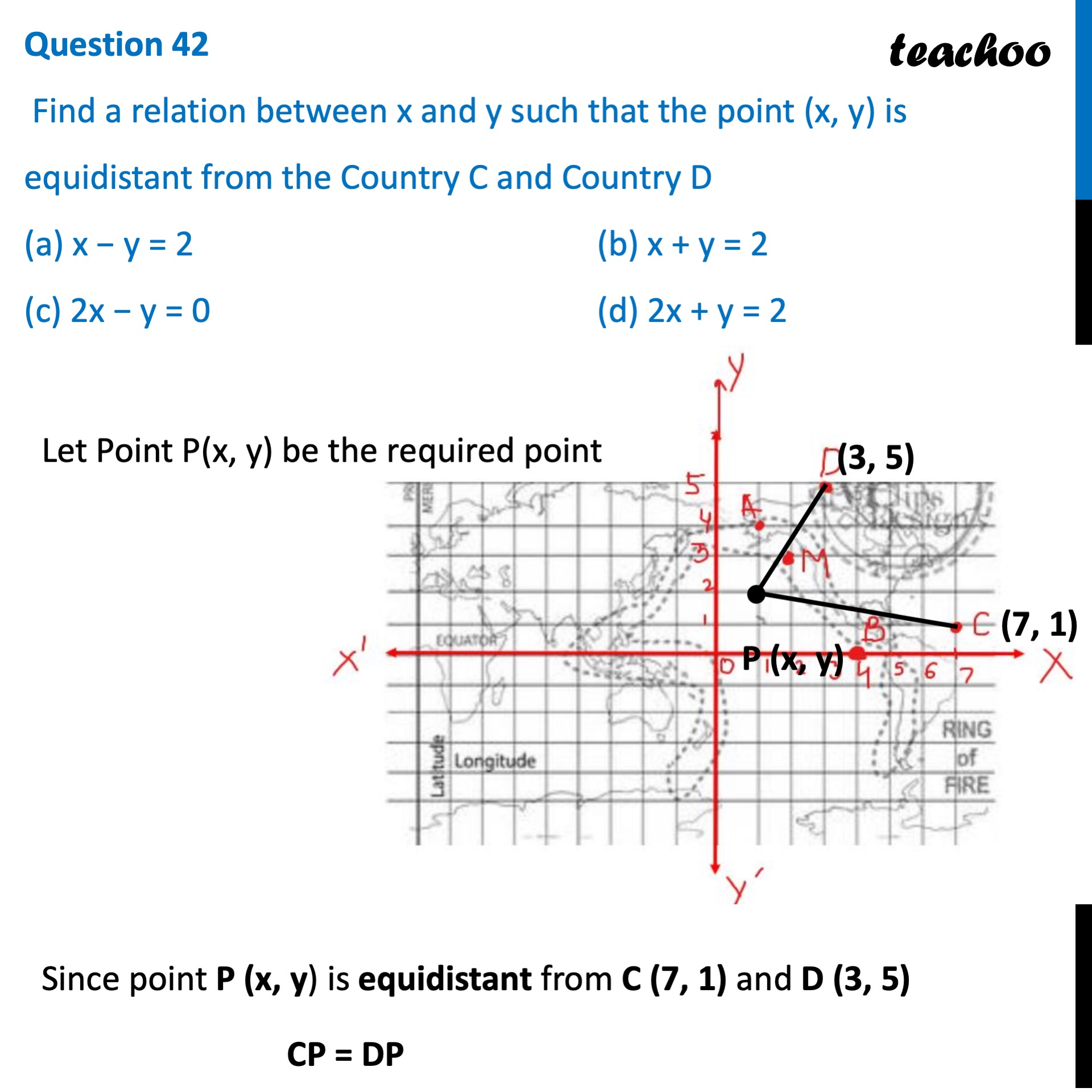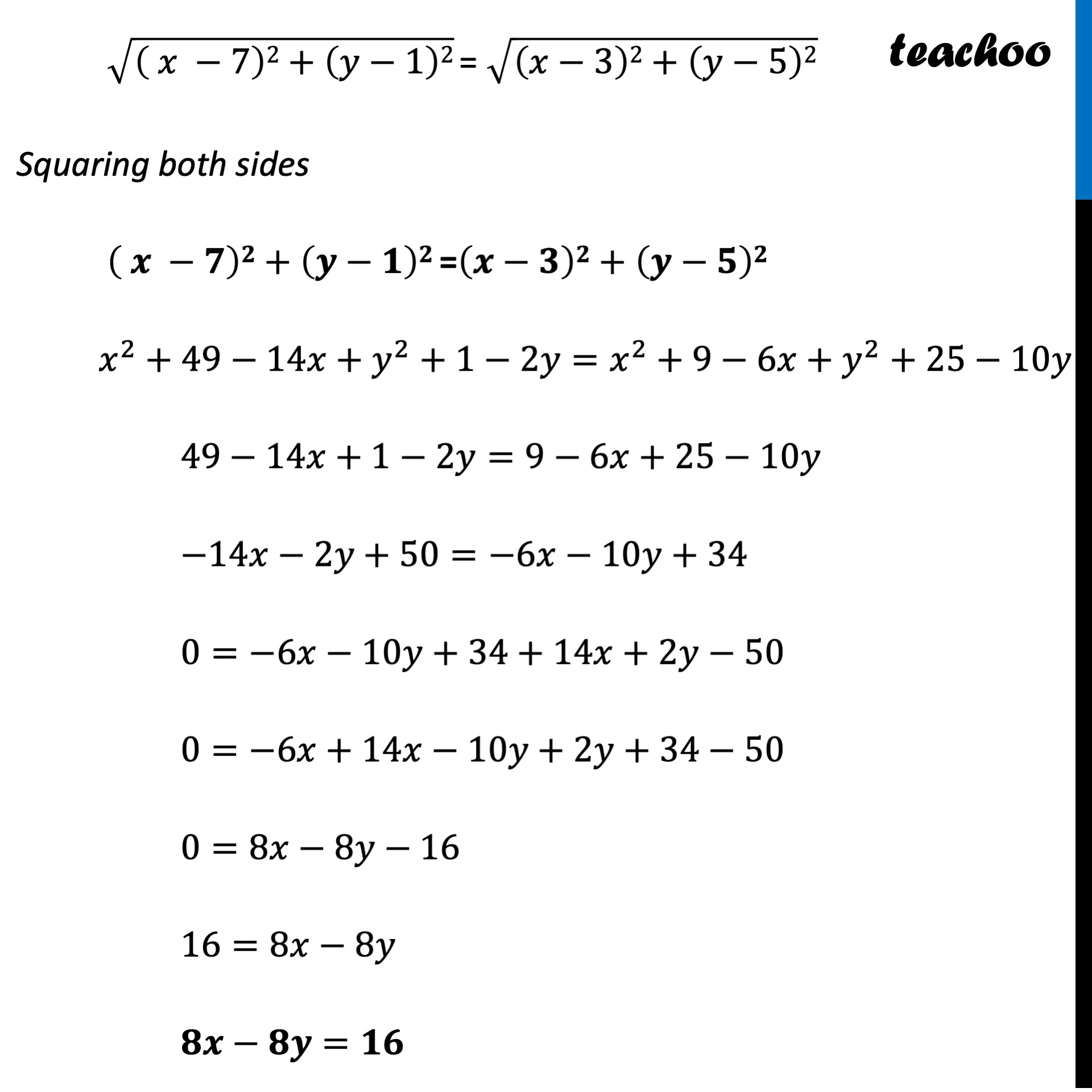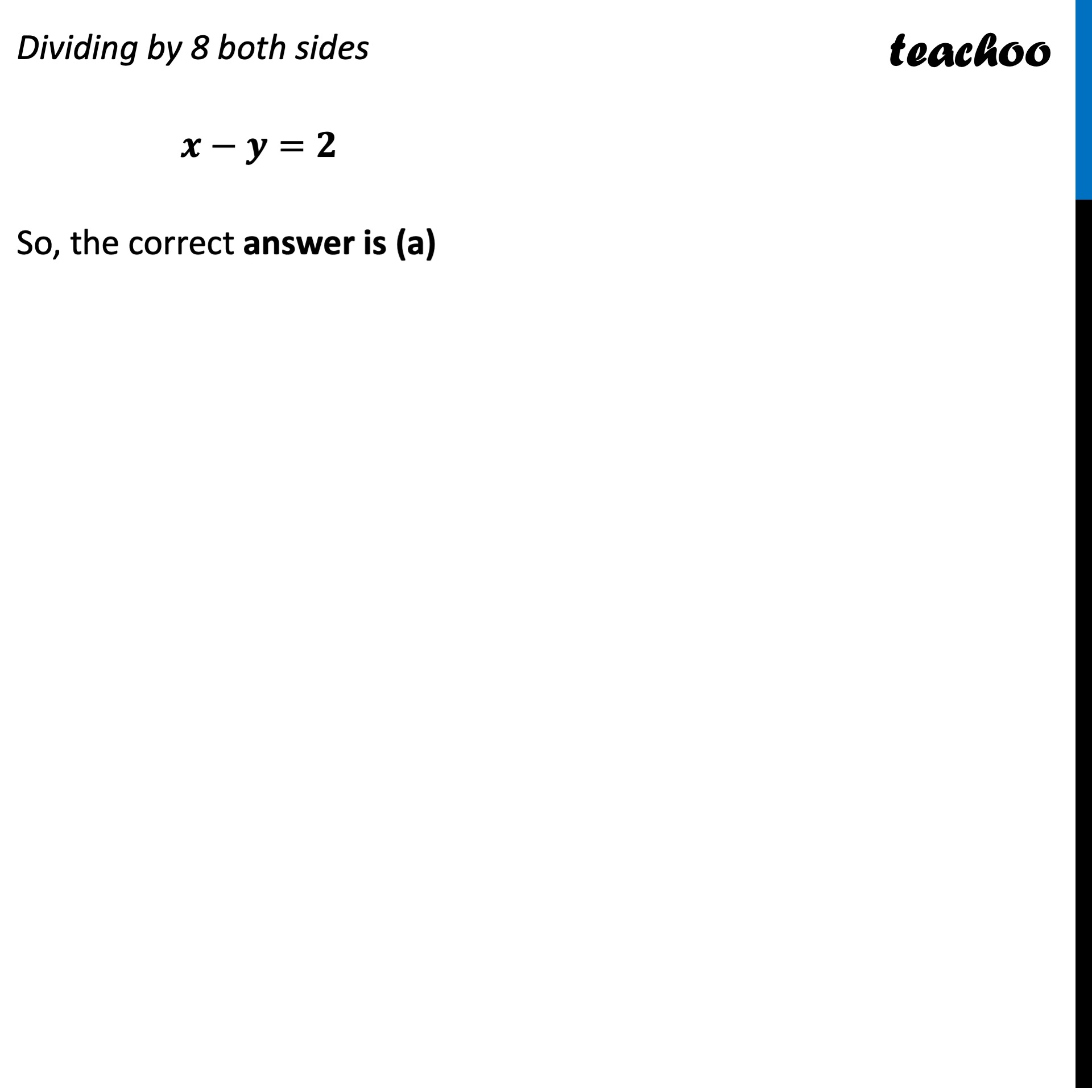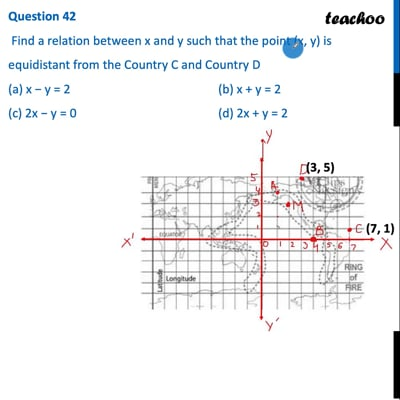CBSE Class 10 Sample Paper for 2022 Boards - Maths Basic [MCQ]

Class 10
Solutions of Sample Papers for Class 10 Boards

## (c) 2x − y = 0   (d) 2x + y = 2This video is only available for Teachoo black users

Introducing your new favourite teacher - Teachoo Black, at only ₹83 per month

### Transcript

Question 42 Find a relation between x and y such that the point (x,y) is equidistant from the Country C and Country D (a) x − y = 2 (b) x + y = 2 (c) 2x − y = 0 (d) 2x + y = 2 Let Point P(x, y) be the required point Since point P (x, y) is equidistant from C (7, 1) and D (3, 5) CP = DP √(( 𝑥 −7)2+(𝑦−1)2) = √((𝑥−3)2+(𝑦−5)2) Squaring both sides ( 𝒙 −𝟕)𝟐+(𝒚−𝟏)𝟐 =(𝒙−𝟑)𝟐+(𝒚−𝟓)𝟐 𝑥^2+49−14𝑥+𝑦^2+1−2𝑦=𝑥^2+9−6𝑥+𝑦^2+25−10𝑦 49−14𝑥+1−2𝑦=9−6𝑥+25−10𝑦 −14𝑥−2𝑦+50=−6𝑥−10𝑦+34 0=−6𝑥−10𝑦+34+14𝑥+2𝑦−50 0=−6𝑥+14𝑥−10𝑦+2𝑦+34−50 0=8𝑥−8𝑦−16 16=8𝑥−8𝑦 𝟖𝒙−𝟖𝒚=𝟏𝟔 Dividing by 8 both sides 𝒙−𝒚=𝟐 So, the correct answer is (a)